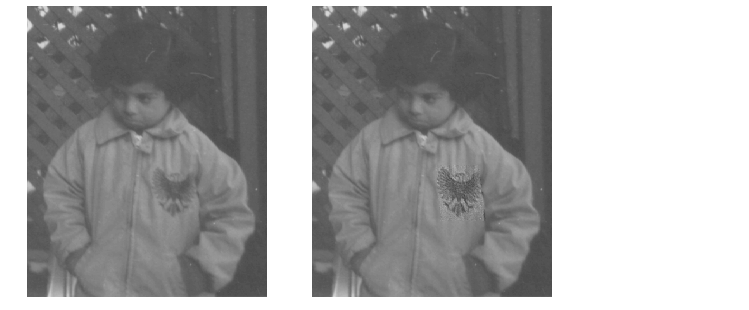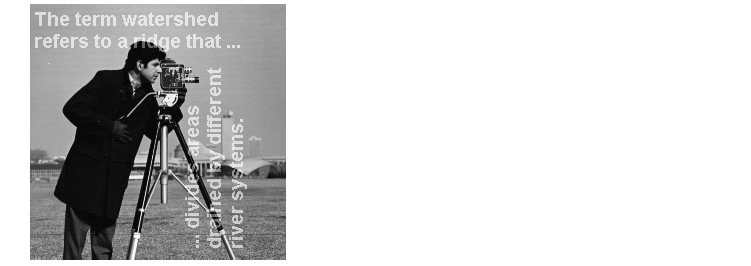Image Processing Toolbox User's GuideFiltering a Region

Filtering a region is the process of applying a filter to a region of interest in an image, where a binary mask defines the region. For example, you can apply an intensity adjustment filter to certain regions of an image.

To filter a region in an image, use the `roifilt2` function. When you call `roifilt2`, you specify an grayscale image, a binary mask, and a filter. `roifilt2` filters the input image and returns an image that consists of filtered values for pixels where the binary mask contains 1's and unfiltered values for pixels where the binary mask contains 0's. This type of operation is called masked filtering.

 Note    `roifilt2` is best suited to operations that return data in the same range as in the original image, because the output image takes some of its data directly from the input image. Certain filtering operations can result in values outside the normal image data range (i.e., [0,1] for images of class `double`, [0,255] for images of class `uint8`, and [0,65535] for images of class `uint16`). For more information, see the reference page for `roifilt2`.

Example: Filtering a Region in an Image

This example uses masked filtering to increase the contrast of a specific region of an image:

• ```I = imread('pout.tif');
```
1. This example uses the mask `BW` created in Selecting a Polygon. The region of interest specified by the mask is the logo on the girl's jacket.

1. Create the filter.
• ```h = fspecial('unsharp');
```
2. Call `roifilt2`, specifying the image to be filtered, the mask, and the filter.
• ```I2 = roifilt2(h,I,BW);
imshow(I)
figure, imshow(I2)```

Image Before and After Using an Unsharp Filter on the Region of Interest

Specifying the Filtering Operation

`roifilt2` also enables you to specify your own function to operate on the region of interest. This example uses the `imadjust` function to lighten parts of an image:

• ```I = imread('cameraman.tif');
```
2. Create the mask. In this example, the mask is a binary image containing text. The mask image must be cropped to be the same size as the image to be filtered.
• ```BW = imread('text.png');
```
3. Create the filter.
• ```f = inline('imadjust(x,[],[],0.3)');
```
4. Call `roifilt2`, specifying the image to be filtered, the mask, and the filter. The resulting image, `I2`, has the text imprinted on it.
• ```I2 = roifilt2(I,mask,f);
imshow(I2)```

Image Brightened Using a Binary Mask Containing TextOther Selection Methods Filling a Region